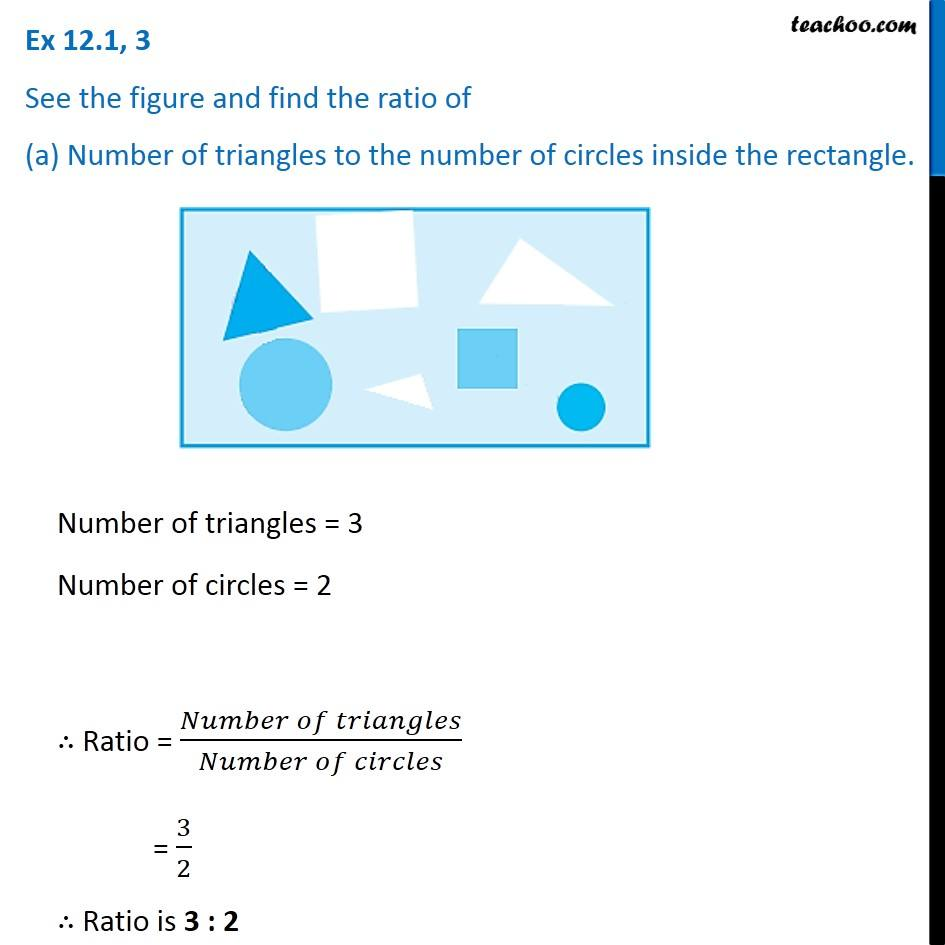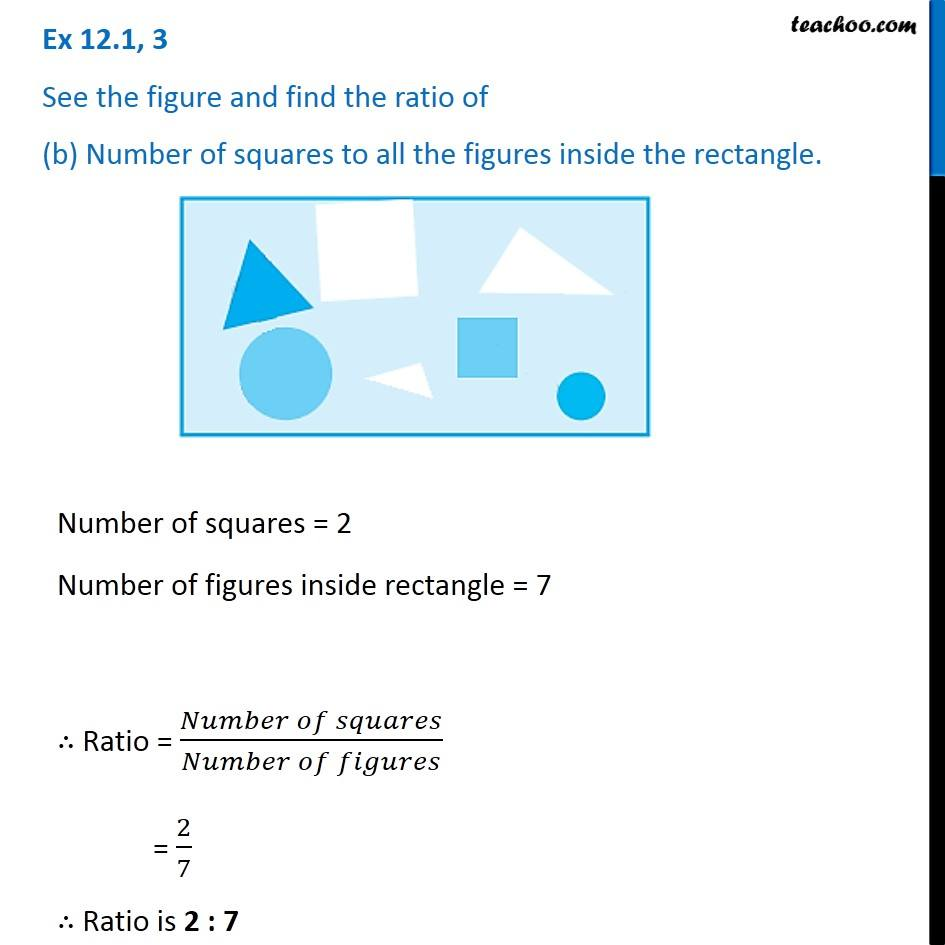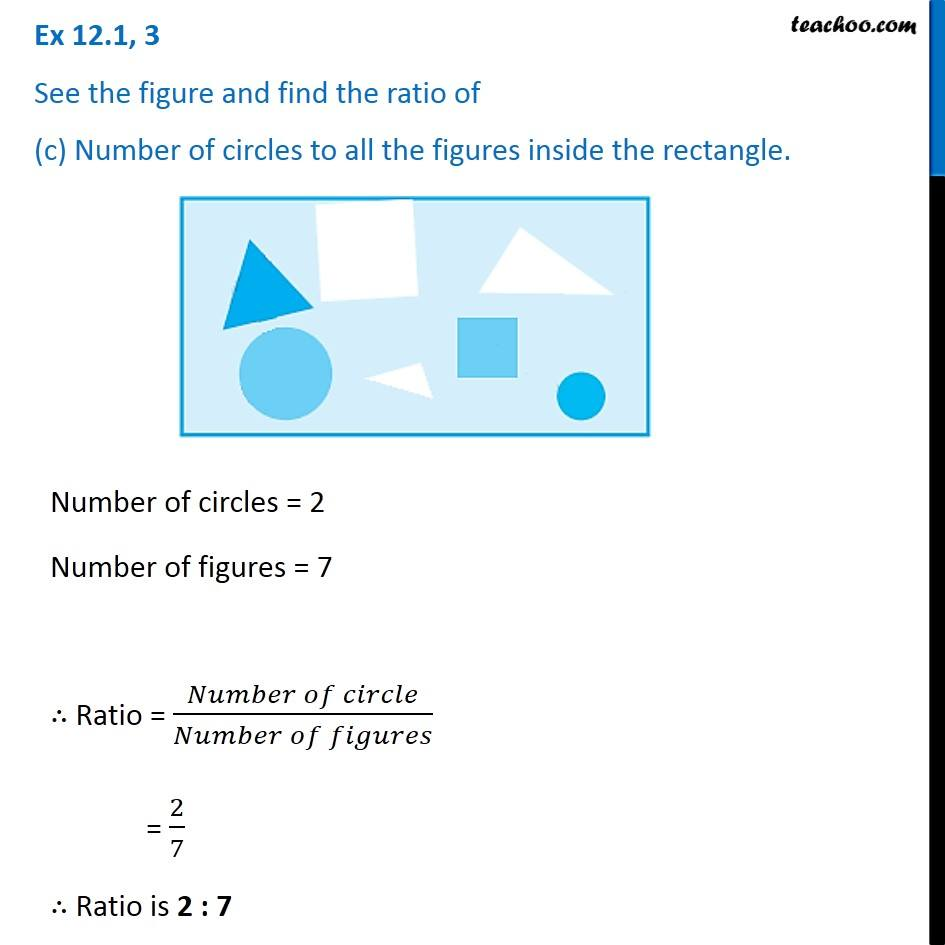Ex 12.1

Chapter 12 Class 6 Ratio and Proportion
Serial order wiseLearn in your speed, with individual attention - Teachoo Maths 1-on-1 Class

### Transcript

Ex 12.1, 3 See the figure and find the ratio of (a) Number of triangles to the number of circles inside the rectangle. Number of triangles = 3 Number of circles = 2 ∴ Ratio = (𝑁𝑢𝑚𝑏𝑒𝑟 𝑜𝑓 𝑡𝑟𝑖𝑎𝑛𝑔𝑙𝑒𝑠)/(𝑁𝑢𝑚𝑏𝑒𝑟 𝑜𝑓 𝑐𝑖𝑟𝑐𝑙𝑒𝑠) = 3/2 ∴ Ratio is 3 : 2 Ex 12.1, 3 See the figure and find the ratio of (b) Number of squares to all the figures inside the rectangle.Number of squares = 2 Number of figures inside rectangle = 7 ∴ Ratio = (𝑁𝑢𝑚𝑏𝑒𝑟 𝑜𝑓 𝑠𝑞𝑢𝑎𝑟𝑒𝑠)/(𝑁𝑢𝑚𝑏𝑒𝑟 𝑜𝑓 𝑓𝑖𝑔𝑢𝑟𝑒𝑠) = 2/7 ∴ Ratio is 2 : 7 Ex 12.1, 3 See the figure and find the ratio of (c) Number of circles to all the figures inside the rectangle.Number of circles = 2 Number of figures = 7 ∴ Ratio = (𝑁𝑢𝑚𝑏𝑒𝑟 𝑜𝑓 𝑐𝑖𝑟𝑐𝑙𝑒)/(𝑁𝑢𝑚𝑏𝑒𝑟 𝑜𝑓 𝑓𝑖𝑔𝑢𝑟𝑒𝑠) = 2/7 ∴ Ratio is 2 : 7